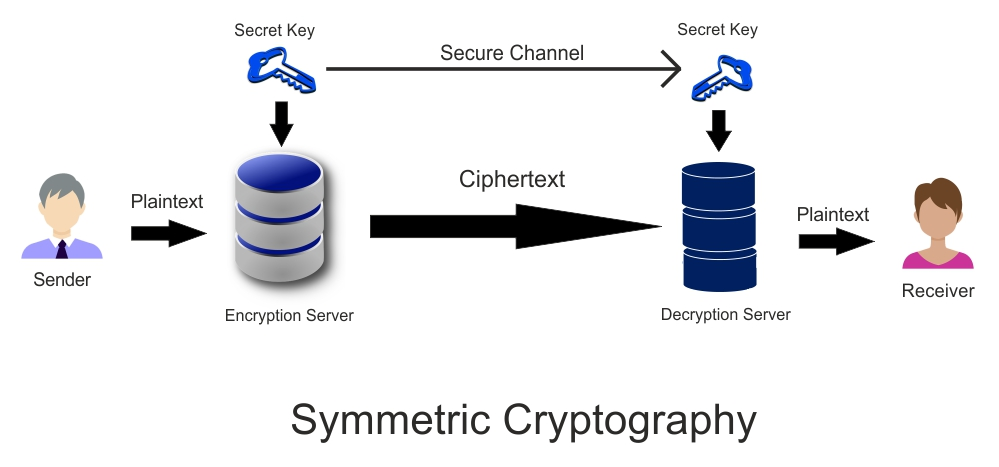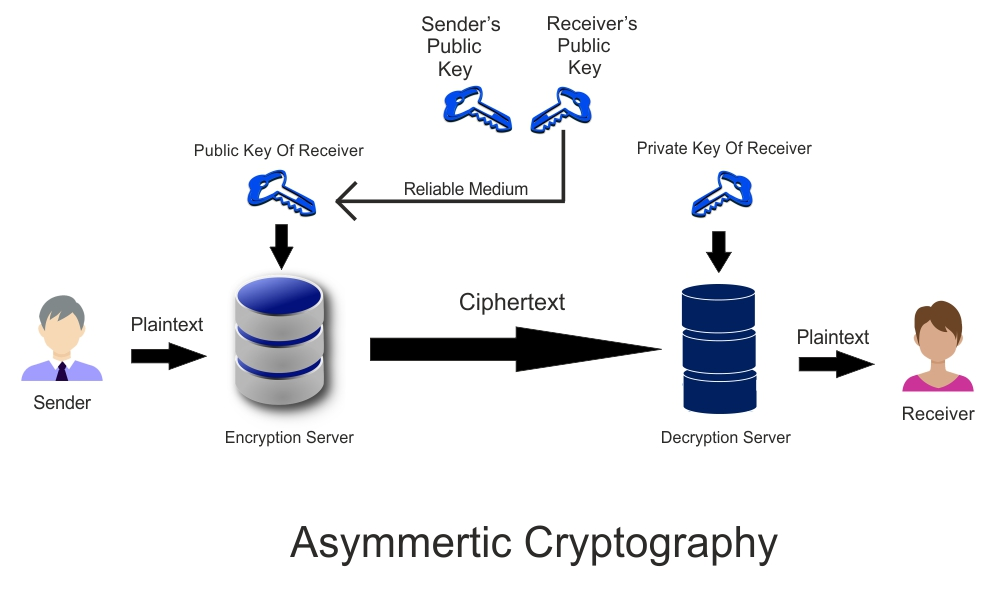# What Is Cryptography | Working Of Cryptography | Cryptography Techniques## Introduction Of Cryptography

Cryptography is very old technique. Egyptian and Roman civilization were using it. There were some designs or symbols which were having specific meaning. Which could be understand by the person who is carrying the message generally.

Later on these designs and symbols have changed into the alphabets. So there were some rule to read these alphabets. These rules works as the Key in cryptography. Caesar Shift Cipher were the famous roman method cryptography.

Caesar Shift Cipher means to disorder the alphabets in a specific rule. So by applying this algorithm no one can not understand until he knows its letter shifting rules. For example,

A B C D E F G H will be replaced with D E F G H I J K

where you can easily understand rule of third is used because each letter is in increasing order of +3. It can also be called as Traditional Cryptography.

So today in this article we will cover what is cryptography, Types Of Cryptography and Working of Cryptography.

## What Is CryptographyCryptography word came from greek work where Kryptos meaning secret and Graphein means writing. So cryptography means secretly written code in very simple language.

Sending of a data securely by using Powerful Mathematical Algorithm with the Encryption KEY is called the Cryptography.

So that it will be useless for the unauthorized person until he have the Decryption KEY. Anyhow if unauthorized person get to know its encryption algorithm but he will not be unable to decrypt the message until he have the Decryption KEY.

Broadly we can divide the Cryptography in two types,

We uses the numbers and letters in this traditional cryptography. Algorithms were not that much complex.

### (2) Modern Cryptography

We uses the binary numbers in modern cryptography. Encryption Algorithms are very complex requires high computational power to decrypt.

Cryptography comes under Cryptology. Because Cryptology have divided into two parts,

### (2) Cryptanalysis

A system which perform the cryptographic function is called the Cryptosystem. To break the Cryptosystem or CIphertext is called the cryptanalysis. The Cryptanalysis denotes the strength of the Cryptography.

## Working Of CryptographyAs shown above in the figure this is basic working of cryptography. There are two parties named as sender and receiver. The original message is called as Plaintext. This Plaintext sends sender in the encryption server. Where encryption algorithm is applied with the secret key and gives encrypted output as Ciphertext.

This Ciphertext is encrypted output which goes from public domain into the decrption server. Where decryption algorithm is applied with the secret key and gives output Plaintext or original message.

We used here term CIPHER if you want to know more about CIPHER in detail then read it in our other article for that,  click here## Types Of Cryptography

There are two types of cryptography described below,

### (1) Symmetric CryptographyA Cryptography System where Encryption Key and Decryption Key are same is known as Symmetric Cryptography.

As shown above in the figure there are two parties Sender and Receiver wants to transfer the data securely with Symmetric Key Cryptography. Before sending the information sender and receiver will decide a Secret Key which will be shared between them via secure connection.

Now our Plaintext will go into the server where Symmetric Algorithm will be applied with the secure key which results Ciphertext. Now this Ciphertext will be transferred via public connection.

At the receiving end the decryption server is established which will receive the Ciphertext and applies then Symmetric Algorithm with the Secure Key which results Plaintext in output for receiver.

Thus Symmetric Cryptography works.

### (2) Asymmetric CryptographyA Cryptography System where Encryption Key and Decryption Key are different is known as Asymmetric Cryptography.

As shown above in the figure there are two parties Sender and Receiver. In this system the sender should have public key of receiver. Which can get from a reliable medium. Now Plaintext will go into the Encryption Server where Asymmetric Algorithm will be applied with Public Key which results CIphertext as output.

This Ciphertext will go to the Decryption Server. Now this decryption server will apply Asymmetric Algorithm on Ciphertext with Private Key which results Plaintext as output.

## Conclusion Of What Is Cryptography

Sending of information or message securely in public domain which becomes useful for authorized users and useless for unauthorized users is called the Cryptography. It is basically changing the Plaintext into the Ciphertext and vice versa. Where Plaintext is a original message while Ciphertext is encrypted message.

A Cryptographic System having same Encryption Key and Decryption Key is known as Symmetric Cryptography whereas having different Encryption Key and Decryption Key is known as Asymmetric Cryptography.

Thus this is all about what is cryptography.

### Know More About    What Is CIpher & Working Of Ciphers

reference : ronald linnerror: Want To Download These Articles ? Go To Books Tab !!!